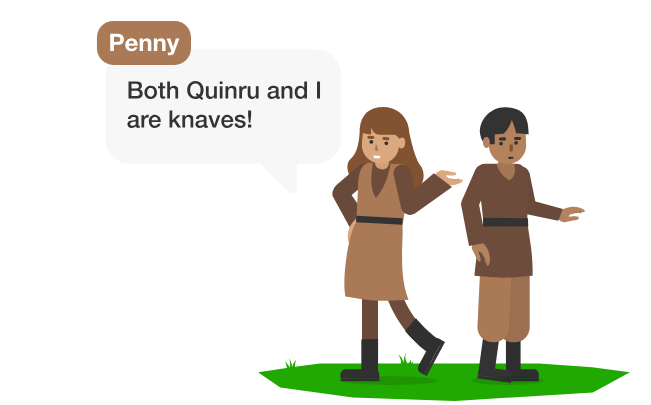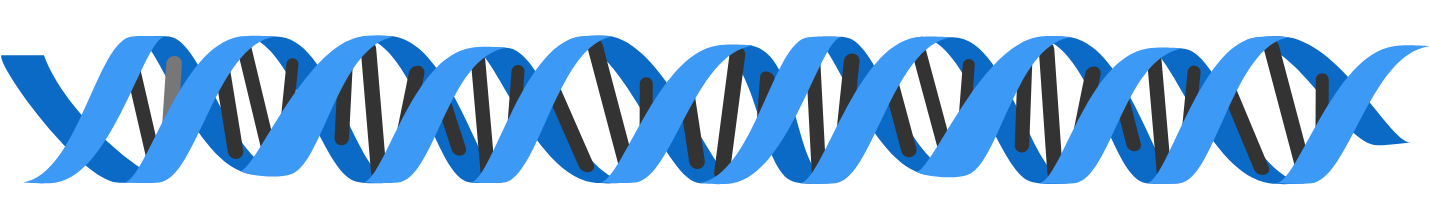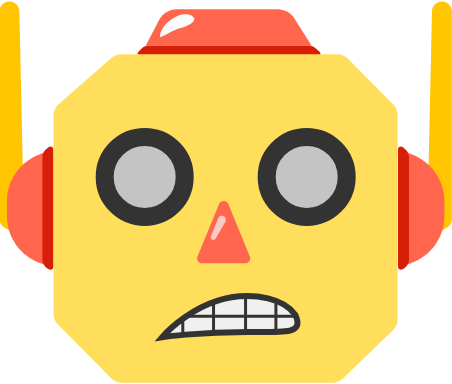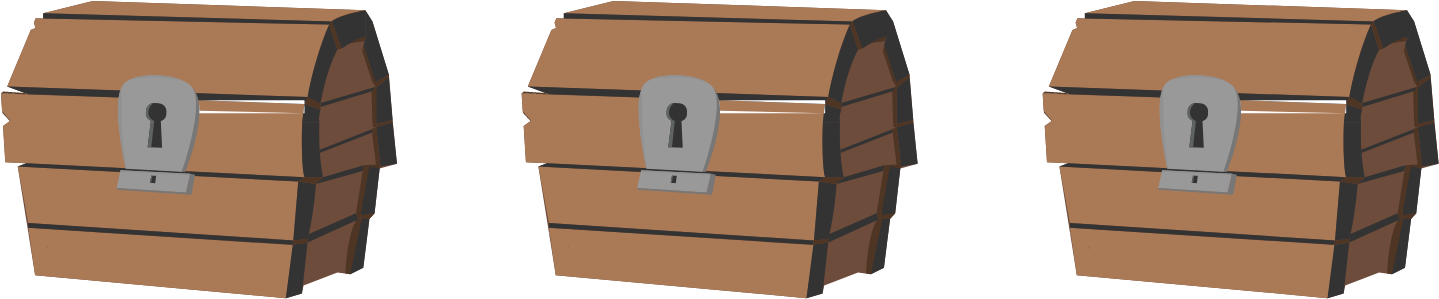### Logic II

Propositional logic was derived for the rigorous exploration of both philosophy and mathematics and has been used to study logic since the $3^\text{rd}$ century BC. We want to teach you this language and show you how to apply it both when solving puzzles and, later in this course, to more serious applications in programming and AI.

First, go ahead and solve the puzzle below without using formal logic, then we'll show you how formal logic works and how you can use it to solve this puzzle and much more.

On the island of knights and knaves (where knights always tell the truth and knaves always lie), you meet two islanders named Penny and Quinru:What are Penny and Quinru?

# Knights and Formal Logic

Now let's dive into the world of formal propositional logic using the previous question as a gateway. Formal logic is a very powerful language for working with logic similarly to how algebra is a powerful language for working with numerical unknowns.

1. When translating a problem into formal logic, we give statements single-letter nicknames known as propositional variables. For example, we might refer to the statement "Penny is a knight." as P.
$\text{ }$
2. The symbol ${\large\equiv}$ is called a biconditional. You can think of it as meaning "if and only if." That is, P $\equiv$ Q means "P is true if and only if Q is true."

Given P $\equiv$ Q, and also knowing Q is false, what must be true about P?

# Knights and Formal Logic$\neg$P is the negation of statement P. It's read as "NOT P."

Using "Penny is a knight" as P, what does $\neg$P indicate?

# Knights and Formal Logic

Similarly to how $+$ and $\times$ are operators in algebra, AND, OR, and $\equiv$ are operators in logic. Truth tables are simply a tabular organization of all of the cases: you fill them in left to right, and the left-most columns enumerate all possible true/false combinations of the propositional variables.

For example, if you have two statements, X, and Y, and each of them might be either true or false, then there are four possible cases to consider.

 X Y X OR Y X $\equiv$ Y $\neg$Y X OR $\neg$Y True True True True False True False True False True False True True False False False False False True True

The Rules
X and Y can be any propositional statements

• X AND Y is true only when both X and Y are true.
• X OR Y is true when one or both of X and Y are true.
• $\neg$X is false when X is true and true when X is false.
• X $\equiv$ Y is true only when X and Y have the same truth values.

In which cases will "X OR $\neg$Y" be true?

# Knights and Formal Logic

Select one or more

Suppose P is the statement "Penny is a knight" and Q is the statement "Quinru is a knight."

How would the statement "Both Quinru and Penny are knaves" be written in formal logic?

(Remember we're using $\neg$ to stand for "NOT.")

# Knights and Formal Logic

Now, here's where it gets a bit tricky! On the island of knights and knaves, knights always speak the truth and knaves always lie. To translate this idea into formal logic, we say:

A statement spoken by an islander is true if and only if the person making the statement is a knight.What formal logic expresses the scenario of Penny saying, "Quinru and I are knaves" in this puzzle?

Remember,

• P stands for the statement "Penny is a knight,"
• Q stands for the statement "Quinru is a knight," and
• the symbol $\equiv$ means "if and only if."

# Knights and Formal Logic

Now that we've written our statement in formal logic, let's solve the puzzle in a way that doesn't resort to exhaustively listing every case.

P $\equiv$ $\neg$P AND $\neg$Q

We're going to attempt a proof by contradiction, where we assume something is true, then show it leads to an impossible scenario.

If we suppose that P is true, $\neg$P AND $\neg$Q (the right side of the biconditional) is also true.

Given $\neg$P AND $\neg$Q is true, how many of the following statements must be true?

$\neg$P

$\neg$Q

# Knights and Formal Logic

In the prior problem, supposing P is true (that is, supposing P is a knight) led to these statements being true.

P

$\neg$P AND $\neg$Q

$\neg$P

$\neg$Q

Notice the first and third lines: P and $\neg$P are true at the same time! That means Penny is both a knight and a knave, which can't happen! So our original assumption of P being true doesn't work: P can't be a knight and must be a knave instead.

Since Penny is a knave, we now know $\neg$P is true and P is false. Let's substitute in our original P $\equiv$ $\neg$P AND $\neg$Q statement:

false $\equiv$ true AND $\neg$Q

This line is enough to get: is Quinru a knight or a knave? (You already worked this out before, but try thinking it through just from the line above.)

# Knights and Formal Logic

Formal propositional logic is in its essence a type of algebra that allows transformation of statements from one form to another. One important use is to systemize and simplify logic problems, and let us turn statements like

Penny says, "It's not true that: if I am a knave then Quinru is a knight."

into statements like

Penny says, "I am a knave and Quinru is a knave."

(Yes, these are equivalent! Which is easier to understand? Which would be easier to make using circuitry, or implement in computer code?)

It lets us handle situations where we might have tens or hundreds or even thousands of variables (perhaps referring to legal codes, or DNA strands), where a truth table would be impractical even by computer.In this course, you'll use formal logic to solve some very challenging puzzles that are really only approachable using the logical tools you just learned, and we'll also go far beyond puzzles, applying formal logic both to create artificial intelligences and to define some of their limits.# Knights and Formal Logic

One last puzzle!

The three treasure chests below each contain either gold coins or silver coins, but not both.

Given the facts below, are the coins in the third chest silver or gold?Known facts:

1) The first and third chest do not contain the same type of coins.
2) The first chest contains gold coins if and only if the second chest contains silver coins and the third chest contains gold coins.

# Knights and Formal Logic

×